# Cold conditioned air at 10°C is flowing inside a 1.5 cm thick square aluminum (k = 240 w/mK) duct...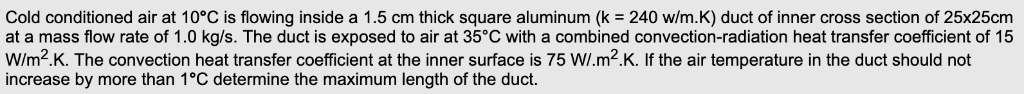Cold conditioned air at 10°C is flowing inside a 1.5 cm thick square aluminum (k = 240 w/mK) duct of inner cross section of 25x25cm at a mass flow rate of 1.0 kg/s. The duct is exposed to air at 35°C with a combined convection-radiation heat transfer coefficient of 15 W/m2K. The convection heat transfer coefficient at the inner surface is 75 W/.m2.K. If the air temperature in the duct should not increase by more than 1°C determine the maximum length of the duct.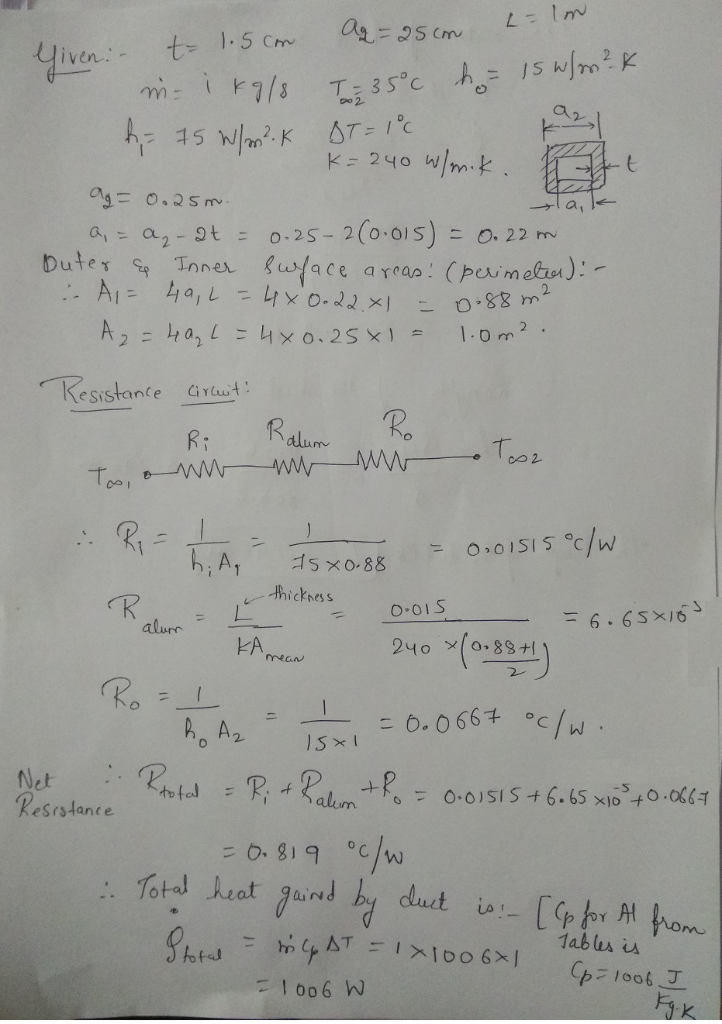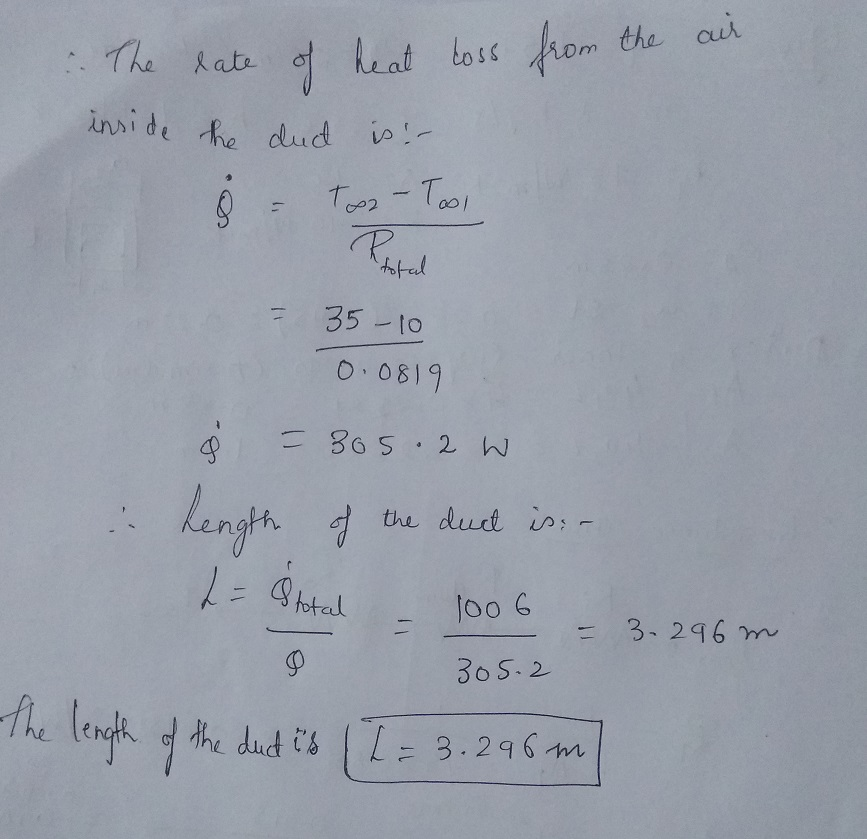Let me know if you have any doubts and please provide feedback by rating.

##### Add Answer of: Cold conditioned air at 10°C is flowing inside a 1.5 cm thick square aluminum (k = 240 w/mK) duct...
Similar Homework Help Questions
• ### 3 Steam at T1c-320°C flows in a cast iron pipe (k = 80 W/m·°C) whose inner...

3 Steam at T1c-320°C flows in a cast iron pipe (k = 80 W/m·°C) whose inner and outer diameters are D1 = 5 cm and = 5.5 cm, respectively. The pipe is covered with 3-cm-thick glass wool insulation with k = 0.05 W/m °C, Heat is lost to the surroundings at Ta=5°C by natural convection and radiation, with a combined heat transfer coefficient of ha = 18 W/m2·°C. Taking the heat transfer coefficient inside the pipe to be hte =...

• ### Problem 3 (30): Steam at Too,1 340 °C flows in a cast iron pipe [k- 80 W/m.°C] whose inner and outer diameter are Di 6 cm and D2 -8 cm, respectively. The pipe is covered with a 4-cm thick glass wool...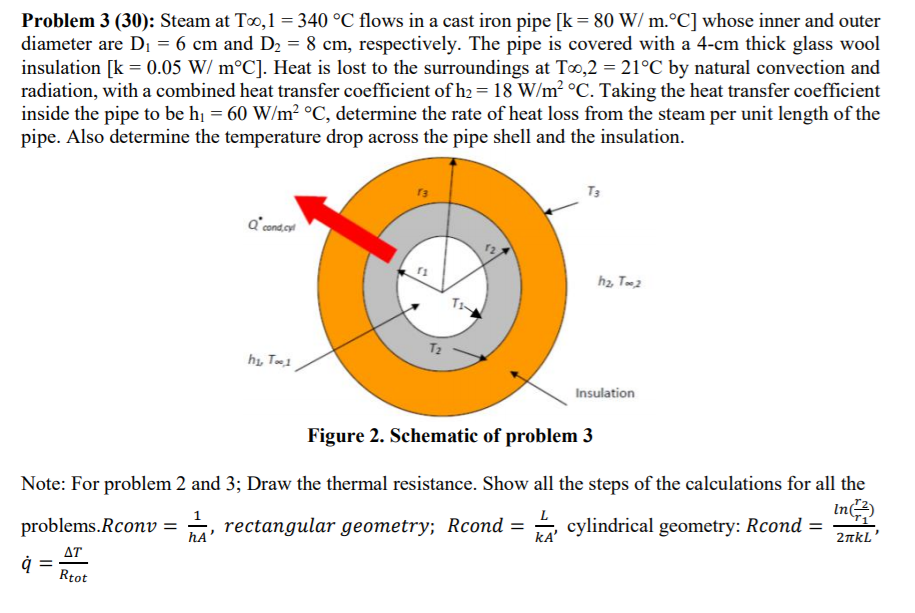Problem 3 (30): Steam at Too,1 340 °C flows in a cast iron pipe [k- 80 W/m.°C] whose inner and outer diameter are Di 6 cm and D2 -8 cm, respectively. The pipe is covered with a 4-cm thick glass wool insulation [k-0.05 W/ m°C]. Heat is lost to the surroundings at Too,2 - 21°C by natural convection and radiation, with a combined heat transfer coefficient of h- 18 W/m2 °C. Taking the heat transfer coefficient inside the pipe to...

• ### A 0.1 m thick brick wall with thermal conductivity 0.66 Wm K-1 is exposed to cold wind on the out...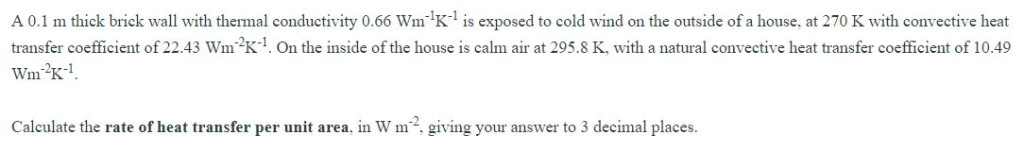A 0.1 m thick brick wall with thermal conductivity 0.66 Wm K-1 is exposed to cold wind on the outside of a house, at 270 K with convective heat transfer coefficient of 22.43 Wm2K1. On the inside of the house is calm air at 295.8 K, with a natural convective heat transfer coefficient of 10.49 Calculate the rate of heat transfer per unit area, in Wm, giving your answer to 3 decimal places A 0.1 m thick brick wall with...

• ### H8-1: An 8-m-internal-diameter spherical tank made of 1.5-cm-thick stainless steel (k-15 W/m K) i...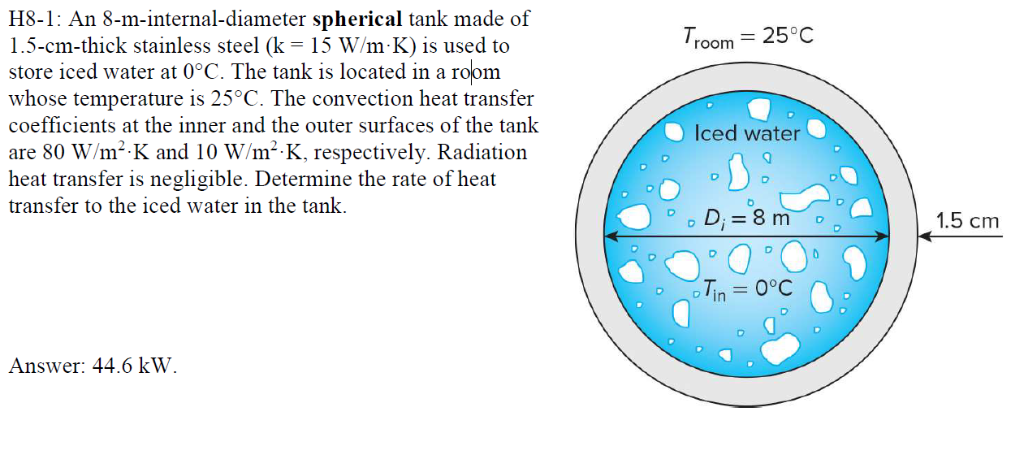H8-1: An 8-m-internal-diameter spherical tank made of 1.5-cm-thick stainless steel (k-15 W/m K) is used to store iced water at 0°C. The tank is located in a room whose temperature is 25°C. The convection heat transfer coefficients at the inner and the outer surfaces of the tank are 80 W/m2 K and 10 W/m2 K, respectively. Radiatior heat transfer is negligible. Determine the rate of heat transfer to the iced water in the tank. room 25°0c T. Iced water 0...

• ### A large wall made of 10-cm-thick solid brick (k = 0.8 W/m.K, p 2000 kg/m3, and p 800 J/kg.K) is o...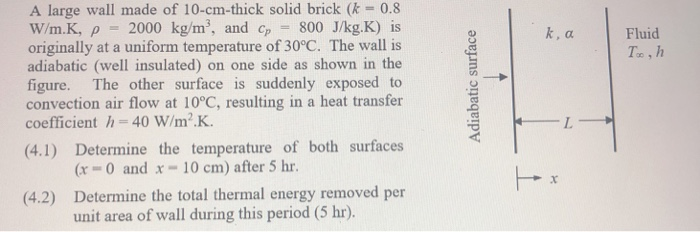Heat transfer question. A large wall made of 10-cm-thick solid brick (k = 0.8 W/m.K, p 2000 kg/m3, and p 800 J/kg.K) is originally at a uniform temperature of 30°C. The wall is adiabatic (well insulated) on one side as shown in the figure. The other surface is suddenly exposed to convection air flow at 10°C, resulting in a heat transfer coefficient h=40W/m2.K. Fluid (4.1) Determine the temperature of both surfaces Gr 0 and x 10 cm) after 5 hr...

• ### Thin-walled aluminum tubes of diameter D = 10 mm are used in the condenser of an air conditioner....

Thin-walled aluminum tubes of diameter D = 10 mm are used in the condenser of an air conditioner. Under normal operating conditions, a convection coefficient of hi = 5000 W/m2 · K is associated with condensation on the inner surface of the tubes, while a coefficient of ho = 100 W/m2 · K is maintained by airflow over the tubes. a. What is the overall heat transfer coefficient if the tubes are unfinned? b. What is the overall heat transfer...

• ### 4. Air at 200 °C is flowing inside rectangular tube (2cm x 6cm cross section) with...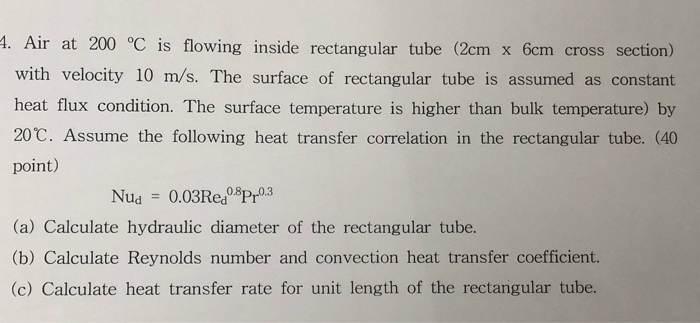4. Air at 200 °C is flowing inside rectangular tube (2cm x 6cm cross section) with velocity 10 m/s. The surface of rectangular tube is assumed as constant heat flux condition. The surface temperature is higher than bulk temperature) by 20 C. Assume the following heat transfer correlation in the rectangular tube. (40 point) 0.03Rea0Pr03 0.81 Nud (a) Calculate hydraulic diameter of the rectangular tube. (b) Calculate Reynolds number and convection heat transfer coefficient. (c) Calculate heat transfer rate for...

• ### 11) Consider a large 5-cm-thick brass plate (k111 W/m-K) in whic uniformly at a rate of 200,000W Im3. One side of th...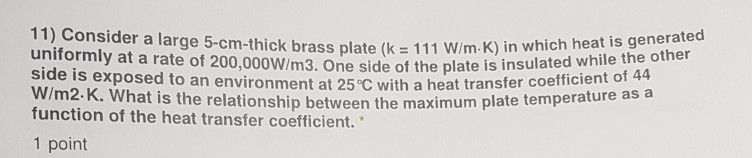11) Consider a large 5-cm-thick brass plate (k111 W/m-K) in whic uniformly at a rate of 200,000W Im3. One side of the plate is insulated while the other exposed to an environment at 25 C with a heat transfer coefficient of What is the relationship between the maximum plate temperature as W/m2.K. function of the heat transfer coefficient." 1 point 11) Consider a large 5-cm-thick brass plate (k111 W/m-K) in whic uniformly at a rate of 200,000W Im3. One side...

• ### this is the full problem. 11.1 An industrial boiler consists of tubes inside of which flow hot combustion gases. Water...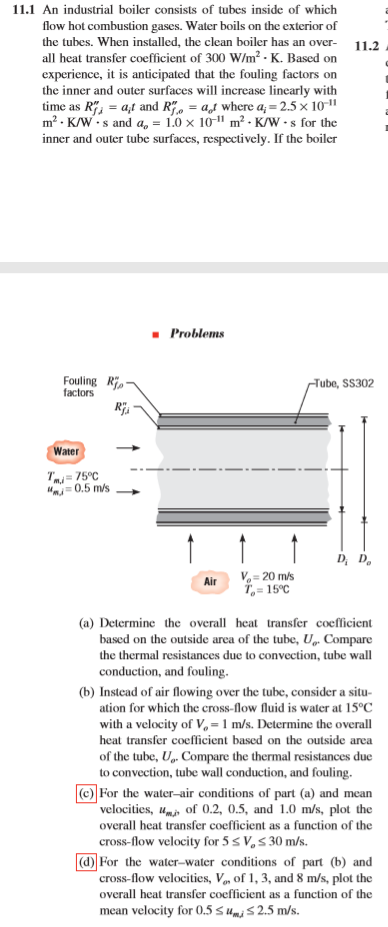this is the full problem. 11.1 An industrial boiler consists of tubes inside of which flow hot combustion gases. Water boils on the exterior of the tubes. When installed, the clean boiler has an over- all heat transfer coefficient of 300 W/m2- K. Based on experience, it is anticipated that the fouling factors on the inner and outer surfaces will increase linearly with time as Rat and R = at where a;= 2.5 x 10-1" m2- K/Ws and a =...

• ### FIGURE P17-56E e develop imensional be used 7Clothing made of several thin la hat kindtrapped air in between, often called ski c layers of fahr clothing, is ly used in cold climates because it is...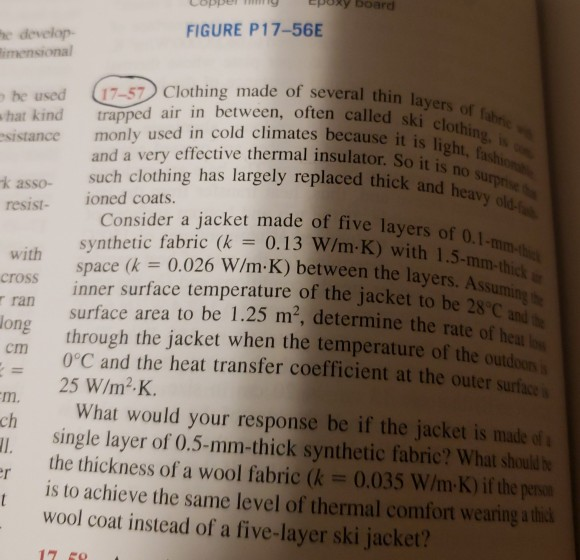FIGURE P17-56E e develop imensional be used 7Clothing made of several thin la hat kindtrapped air in between, often called ski c layers of fahr clothing, is ly used in cold climates because it is light and a very effective thermal insulator. So it is n esistance monly used in cold climates because iti k asso such clothing has largely replaced thick a resist- ioned coats. hick and heavy old Consider a jacket made of five layers of o synthetic...

Need Online Homework Help?